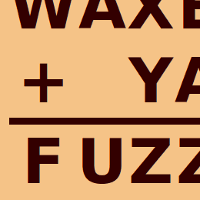# Some Cryptarithm Puzzles

### ... also known as alphametics or crypt-arithmetics

By

Cryptarithm puzzles are fun, challenging puzzles good for anyone from age 10 to 100 or more.  In a cryptarithm puzzle, a normal arithmetic calculation has had all the digits replaced by letters of the alphabet. The aim of the puzzle is to find out what digit each letter represents. If you like cryptarithms, you might also like multiplication strikeout.

Cryptarithms are sometimes called crypt-arithmetic puzzles (from the words cryptic and arithmetic), or alphametics (from alphabet and arithmetic) or letter arithmetic or verbal arithmetic. When I decided to make a cryptarithms game for this website, I wrote a computer program to generate them. To my surprise, it started producing millions and millions of puzzles - far too many for this website!

I've picked out a few of the best ones put them here, but if you want more, I've put the other millions and millions on a separate website - Cryptarithmania.

In the meantime, scroll down to enjoy the ones I've picked out here!

Remember that for each puzzle, these rules apply :

• Each letter corresponds to a unique digit from 0 to 9 - different letters correspond to different digits.
• No number in the sum has a leading 0.
• Each puzzle has a single unique solution
Now for a few math-themed puzzles. This one tells you that if you keep a positive attitude to math, you'll find it a treat!

 E A G E R + M A T H T R E A T

If you really get math into your heart, it's like a poem or a beautiful song...

 H E A R T + M A T H R H Y M E

Sometimes, to get good at math, you just need to practice and practice until it becomes a habit...

 M A T H + M A T H H A B I T

... and finally, when you become mighty at math, you'll find it a lot less stressful!

 M I G H T + M A T H H A P P Y

Here's a few tougher puzzles, involving much bigger numbers. This first one reminds me of the story of Mary Poppins...

 A L T I T U D E + R E M E D I E D U M B R E L L A

Perhaps a person who fixes bedding material?

 C O R R E C T S + R E J E C T E D M A T T R E S S

Life is full of surprises - as this puzzle explains, it's likely that we will enjoy multiple surprises...

 M U L T I P L E + S U R P R I S E L I K E L I E R

Well, that's all for now. Remember to visit the site where I've put the rest of the cryptarithms I found!